# HSPT Math : How to simplify expressions

## Example Questions

← Previous 1

### Example Question #1 : How To Simplify Expressions

You are given that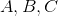are whole numbers.

Which of the following is true ofifandare both odd?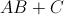is always odd if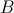is odd, and always even ifis even.is always odd ifis even, and always even ifis odd.is always odd.

None of the other statements are true.is always even.is always odd ifis even, and always even ifis odd.

Explanation:

Ifis odd, then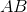is odd, since the product of two odd whole numbers must be odd. When the odd numberis added, the result,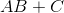, is even, since the sum of two odd numbers must be even.

Ifis even, thenis even, since the product of an odd number and an even number must be even. When the odd numberis added, the result,, is odd, since the sum of an odd number and an even number must be odd.

### Example Question #1 : How To Simplify Expressions

Simplify the expression: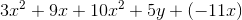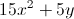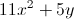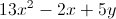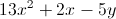Explanation:

Combine all the like terms.

Theterms can be combined together, which gives you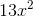.

When you combine theterms together, you get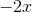.

There is only oneterm so it doesn't get combined with anything. Put them all together and you get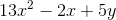.

### Example Question #100 : Algebra

Simplify the following expression: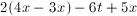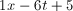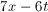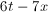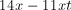Explanation:First distribute the 2: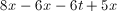Combine the like terms:### Example Question #1 : How To Simplify Expressions

Simplify the expression: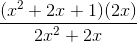x + 1

2x

x+ 2x + 1

2x + 1

x

x + 1

Explanation:

Factor out a (2x) from the denominator, which cancels with (2x) from the numerator. Then factor the numerator, which becomes (+ 1)(+ 1), of which one of them cancels and you're left with (+ 1).

### Example Question #3 : How To Simplify Expressions

Simplify the following expression: x3 - 4(x2 + 3) + 15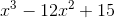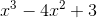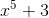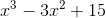Explanation:

To simplify this expression, you must combine like terms. You should first use the distributive property and multiply -4 by x2 and -4 by 3.

x3 - 4x2 -12 + 15

You can then add -12 and 15, which equals 3.

You now have x3 - 4x2 + 3 and are finished. Just a reminder that x3 and 4x2 are not like terms as the x’s have different exponents.

### Example Question #2 : How To Simplify Expressions

Simplify the following expression:

2x(x2 + 4ax – 3a2) – 4a2(4x + 3a)

–12a3 – 14a2x + 2x3

12a– 16a2x + 8ax2 + 2x3

–12a– 14ax2 + 2x3

12a– 22a2x + 8ax2 + 2x3

–12a– 22a2x + 8ax2 + 2x3

–12a– 22a2x + 8ax2 + 2x3

Explanation:

Begin by distributing each part:

2x(x2 + 4ax – 3a2) = 2x * x2 + 2x * 4ax – 2x * 3a2 = 2x3 + 8ax2 – 6a2x

The second:

–4a2(4x + 3a) = –16a2x – 12a3

Now, combine these:

2x3 + 8ax2 – 6a2x – 16a2x – 12a3

The only common terms are those with a2x; therefore, this reduces to

2x3 + 8ax2 – 22a2x – 12a3

This is the same as the given answer:

–12a– 22a2x + 8ax2 + 2x3

### Example Question #1 : How To Simplify Expressions

Which of the following does not simplify to?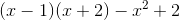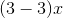All of these simplify to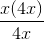Explanation:

5x – (6x – 2x) = 5x – (4x) = x

(x – 1)(x + 2) - x2 + 2 = x2 + x – 2 – x2 + 2 = x

x(4x)/(4x) = x

(3 – 3)x = 0x = 0

### Example Question #2 : How To Simplify Expressions

Simplify: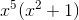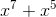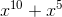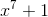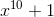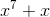Explanation:

In order to simplify this expression, distribute and multiply the outer term with the two inner terms.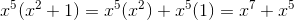### Example Question #4 : How To Simplify Expressions

Simplify: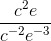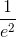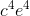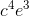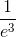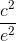Explanation:

When the same bases are multiplied, their exponents can be added.  Similarly, when the bases are divided, their exponents can be subtracted.  Apply this rule for the given problem.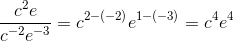### Example Question #3 : How To Simplify Expressions

Simplify: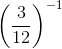Explanation:

To simplify this expression, reduce the term inside the parenthesis.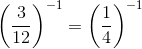Rewrite the negative exponent as a fraction.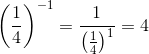← Previous 1